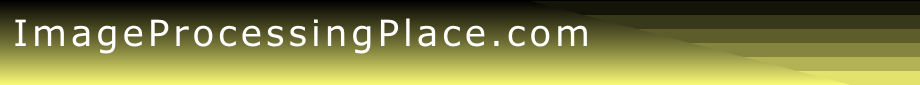Tutorials

The following table summarizes the tutorials available in this site.  The entries in this table are not organized by topic. Use the search feature in your browser to search the table for specific topics such as, for example, MATLAB.

 No. Description Action 000 Review Material (Brief tutorials on probability, linear algebra, and linear systems for readers of Digital Image Processing (all editions). Download 001 Labeling Connected Components (Section 2.4.3 of the 1992 ed. of the DIP book). 002 Relations, Equivalence, and Transitive Closure (Section 2.4.4 of the 1992 ed. of the DIP book). 003 Mask Processing Using an ALU (Arithmetic/Logic Unit) (Section 2.4.6 of the 1992 ed. of the DIP book). 004 Imaging Geometry (perspective transformations, camera modeling, camera calibration, and stereo imaging) (Sec. 2.5 of the 1992 ed. of the DIP book). 005 Basics of Photographic Film (Section 2.6 of the 1992 ed. of the DIP book). 006 RGB/HSI Convertion Equations (Derivations) (From Section 4.6 of the 1992 ed. of the DIP book). 007 Basics of Calculus (Visual Calculus: A visual approach to the fundamentals of calculus). 008 Wavelets (An introduction by Amara Graps ). 009 Wavelets (An ebook by Charles K. Chui--Contains a Wavelet Toolware). 010 MATLAB Tutorials (A list of online sites offering MATLAB tutorials). On the Web 011 MATLAB Tutorial on Matrix Manipulation (An intro. on how to manipulate matrices [images] in MATLAB). 012 Mathematics, Probability, and Related Topics (MathWorld: An excellent resource on math and probability). 013 Probability (Xycoon: Contains a long list of PDFs, CDFs, and random number generating functions). 014 Contour Tracing (An animated discussion of contour tracing, including the Moore neighbor tracing algorithm.)  Contributed by Ms. Abeer George Ghuneim. 015 Overview of Image Processing (Introduction by Bryan-Mac-Namee). 016 Image Processing Overview and  Examples (by B. Girod & G. Wetzstein). 017 Image Processing Fundamentals (I. Young, J. Gerbrands, & L. van Vliet) 018 MATLAB Array Manipulation Tips and Tricks (Very useful collection of array manipulation approaches). Written by Prof. Peter J. Acklam, University of Oslo. 019 A Graphical Illustration of Filtering in the Spatial and Frequency Domains. 020 The Joy of Visual Perception-A Web Book (A comprehensive overview of visual perception, including an index of term definitions).  Written by Professor Peter K. Kaiser, York University. On the web 021 Finding Edges and Lines in Images (Link to John F. Canny's Master's Thesis). This is a large document that takes a while to download. 022 Fuzzy Image Processing 023 L. A. Zadeh's Original Papers on Fuzzy Logic (Well written and definitely worth reading). 024 Algebraic Approach to Image Restoration  (Chapter 5 from the 1992 edition of the book). 025 Image Segmentation and Mathematical Morphology (A short tutorial by Dr. Serge Beucher). 026 Tutorial Presentation on Mathematical Morphology (A PowerPoint presentation by Prof. John Goutsias of the Johns Hopkins University). 027 Gaussian Prob. Density Functions: Properties and Error Characterization (by Dr. Maria Isabel Ribeiro, Inst. Superior Tco, Lisboa, Portugal). 028 cybecollege.com (An interesting site dealing with various aspects of TV and printing technologies,  including historical overviews in areas such as TV cameras, principles of color, and other imaging topics of general interest). 029 All About Pixel Colors (A series of posts by Dr. Steve Eddins that discuss how MATLAB associates matrix values with specific screen pixel colors). 030 Digital Watermarking (by Melinos Averkiou. Contains MATLAB code)) 031 Basic Principles of CT Scanning (An intuitive introduction to CT scanning.) 032 CTSim―Computed Tomography Simulator (A well-documented, open source software package available for Unix and Windows.) 033 Digital Subtraction Angiography (An introductory slide presentation.) 034 Face Recognition (Link to a web site dedicated to face recognition.) 035 Image Fusion (A slide tutorial by Dr.-Ing Michael Heizmann.) 036 Digital Signal Processing (DSP) Tutorial (A good introduction.) 037 Digital Signal Interpolation Tutorial (A good introduction.) 038 Algorithms for Recognizing 2-D Shapes (A tutorial by A. Ashbrook & N. A. Thacker) 039 The Watershed Transform: Strategies for Image Segmentation (Written by Dr. Steve Eddins from the MathWorks). 040 Matrix Indexing in MATLAB (A good introduction with several examples. Written by Steve Eddins and Loren Shure from the MathWorks). 041 MATLAB demos (Examples of how to use MATLAB). 042 MATLAB Image Processing Toolbox demos (Examples of how to use the Image Processing Toolbox). 043 Introduction to MATLAB (by David Houcque, Northwestern University). 044 MATLAB documentation (Detailed MATLAB documentation). 045 Image Processing Toolbox documentation (Detailed documentation on the Toolbox). 046 Steve Eddins' blog on image processing.  Excellent discussion and tutorials on image processing concepts and algorithms, all within the context of MATLAB. 047 A Survey of Image Registration Techniques 048 Data Clustering (Papers and MATLAB code) 049 Image Noise (A slide presentation). 050 Face Hallucination (Theory & Practice--By Liu, Shum, and Freeman). 051 Image Mosaicing (An Introduction by Sevket Gumustekin). 052 Image Motion Compensation (A tutorial by E. D. Fasse, Univ. of Arizona). 053 Generating Synthetic Images (Steve Eddins showing how to use simple math on pixel and coordinate values to generate synthetic test images.) 054 Fingerprint recognition (A short PDF introduction with references). 055 Formulas for color-space conversions 056 A gentle introduction to MATLAB 057 Deep Convolutional Neural Networks (R. C. Gonzalez IEEE Article) View/Download